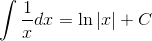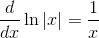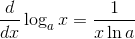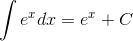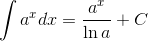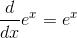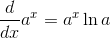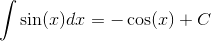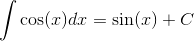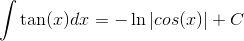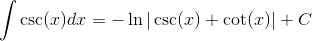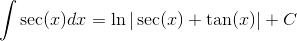### Day 72 - Differential Equations - 12.04.14

• Unit 5 Test on Friday!

Bell Ringer
• Unit 5 Problems
1. Verify that the following has an inverse: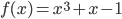. If it exists, find the derivative of the inverse at x = -1.

1. No inverse exists.

2. 1

3. 0

4. -1

5. none of the above

1.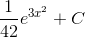2.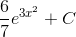3.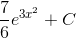4.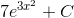5.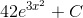2.3.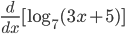Review

Lesson

Exit Ticket
• Posted on the board at the end of the block.
Lesson Objective(s)
• How are differential equations solved using integration and separation of variables?

#### In-Class Help Requests

Standard(s)
• APC.9

• Apply formulas to find derivatives.

• Includes:

• derivatives of algebraic, trigonometric, exponential, logarithmic, and inverse trigonometric functions

• derivations of sums, products, quotients, inverses, and composites (chain rule) of elementary functions

• derivatives of implicitly defined functions

• higher order derivatives of algebraic, trigonometric, exponential, and logarithmic, functions

Math
ematical Practice(s)
• #1 - Make sense of problems and persevere in solving them
• #2 - Reason abstractly and quantitatively
• #5 - Use appropriate tools strategically
• #6 - Attend to precision
• #8 - Look for and express regularity in repeated reasoning

Past Checkpoints
• Differentiation of the Natural Logarithmic Function
• Integration with the Natural Logarithmic Function
• Exponential Functions: Differentiation and Integration
• Bases Other Than e: Differentiation and Integration
• Inverse Functions